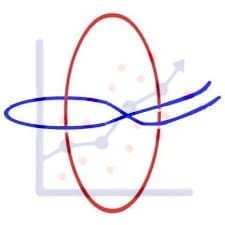Get inspired by the success stories of our students in IIT JAM MS, ISI  MStat, CMI MSc Data Science.  Learn More

# ISI MStat PSB 2012 Problem 6 | Tossing a biased coinThis is a very beautiful sample problem from ISI MStat PSB 2012 Problem 6 based on Conditional probability . Let's give it a try !!

## Problem- ISI MStat PSB 2012 Problem 6

There are two biased coins - one which has probability $1 / 4$ of showing
heads and $3 / 4$ of showing tails, while the other has probability $3 / 4$ of showing heads and $1 / 4$ of showing tails when tossed. One of the two coins is chosen at random and is then tossed 8 times.

(a) Given that the first toss shows heads, what is the probability that in the next 7 tosses there will be exactly 6 heads and 1 tail?
(b) Given that the first toss shows heads and the second toss shows tail, what is the probability that the next 6 tosses all show heads?

## Prerequisites

Basic Counting Principle

## Solution :

Let , $A_1$: Coin with probability of Head 1/4 and Tail 3/4 is chosen
$A_2$ : Coin with probability of Head 3/4 and Tail 1/4 is chosen
B :first toss shows heads and the next 7 tosses there will be exactly 6 heads and 1 tail .
C : the first toss shows heads and the second toss shows tail and the next 6 tosses all show heads .

(a) $P(B)=P(B|A_1)P(A_1) + P(B|A_2)P(A_2)$

Now , $P(B|A_1)= \frac{1}{4} \times {7 \choose 1} \times (\frac{1}{4})^{6} \times \frac{3}{4}$

Since , first toss is head so it can occur by coin 1 with probability 1/4 and out of next 7 tosses we can choose 6 where head comes and this occurs with probability ${7 \choose 1} \times (\frac{1}{4})^{6} \times \frac{3}{4}$

Similarly we can calculate $P(B|A_2)$ and $P(A_1)=P(A_2)= 1/2$ the probability of choosing any one coin out of 2 .

Therefore , $P(B)=P(B|A_1)P(A_1) + P(B|A_2)P(A_2)$

= $\frac{1}{4} \times {7 \choose 1} \times\left(\frac{1}{4}\right)^{6} \times \frac{3}{4} \times\frac{1}{2}+\frac{3}{4} \times {7 \choose 1} \times\left(\frac{3}{4}\right)^{6} \times \frac{1}{4} \times \frac{1}{2}$

(b) Similarly like (a) we get ,

$P(C)= \frac{1}{4} \times \frac{3}{4} \times (\frac{1}{4})^{6} \times \frac{1}{2}+\frac{3}{4} \times \frac{1}{4} \times\left(\frac{3}{4}\right)^{6} \times \frac{1}{2}$ .

Here we don't need to choose any thing as all the outcomes of the toss are given we just need to see for two different coins .

## Food For Thought

There are 10 boxes each containing 6 white and 7 red balls. Two different boxes are chosen at random, one ball is drawn simultaneously at random from each and transferred to the other box. Now a box is again chosen from the 10 boxes and a ball is chosen from it.Find out the probability of the ball being white.

## Subscribe to Cheenta at Youtube

This is a very beautiful sample problem from ISI MStat PSB 2012 Problem 6 based on Conditional probability . Let's give it a try !!

## Problem- ISI MStat PSB 2012 Problem 6

There are two biased coins - one which has probability $1 / 4$ of showing
heads and $3 / 4$ of showing tails, while the other has probability $3 / 4$ of showing heads and $1 / 4$ of showing tails when tossed. One of the two coins is chosen at random and is then tossed 8 times.

(a) Given that the first toss shows heads, what is the probability that in the next 7 tosses there will be exactly 6 heads and 1 tail?
(b) Given that the first toss shows heads and the second toss shows tail, what is the probability that the next 6 tosses all show heads?

## Prerequisites

Basic Counting Principle

## Solution :

Let , $A_1$: Coin with probability of Head 1/4 and Tail 3/4 is chosen
$A_2$ : Coin with probability of Head 3/4 and Tail 1/4 is chosen
B :first toss shows heads and the next 7 tosses there will be exactly 6 heads and 1 tail .
C : the first toss shows heads and the second toss shows tail and the next 6 tosses all show heads .

(a) $P(B)=P(B|A_1)P(A_1) + P(B|A_2)P(A_2)$

Now , $P(B|A_1)= \frac{1}{4} \times {7 \choose 1} \times (\frac{1}{4})^{6} \times \frac{3}{4}$

Since , first toss is head so it can occur by coin 1 with probability 1/4 and out of next 7 tosses we can choose 6 where head comes and this occurs with probability ${7 \choose 1} \times (\frac{1}{4})^{6} \times \frac{3}{4}$

Similarly we can calculate $P(B|A_2)$ and $P(A_1)=P(A_2)= 1/2$ the probability of choosing any one coin out of 2 .

Therefore , $P(B)=P(B|A_1)P(A_1) + P(B|A_2)P(A_2)$

= $\frac{1}{4} \times {7 \choose 1} \times\left(\frac{1}{4}\right)^{6} \times \frac{3}{4} \times\frac{1}{2}+\frac{3}{4} \times {7 \choose 1} \times\left(\frac{3}{4}\right)^{6} \times \frac{1}{4} \times \frac{1}{2}$

(b) Similarly like (a) we get ,

$P(C)= \frac{1}{4} \times \frac{3}{4} \times (\frac{1}{4})^{6} \times \frac{1}{2}+\frac{3}{4} \times \frac{1}{4} \times\left(\frac{3}{4}\right)^{6} \times \frac{1}{2}$ .

Here we don't need to choose any thing as all the outcomes of the toss are given we just need to see for two different coins .

## Food For Thought

There are 10 boxes each containing 6 white and 7 red balls. Two different boxes are chosen at random, one ball is drawn simultaneously at random from each and transferred to the other box. Now a box is again chosen from the 10 boxes and a ball is chosen from it.Find out the probability of the ball being white.

## Subscribe to Cheenta at Youtube

This site uses Akismet to reduce spam. Learn how your comment data is processed.

### Knowledge Partner